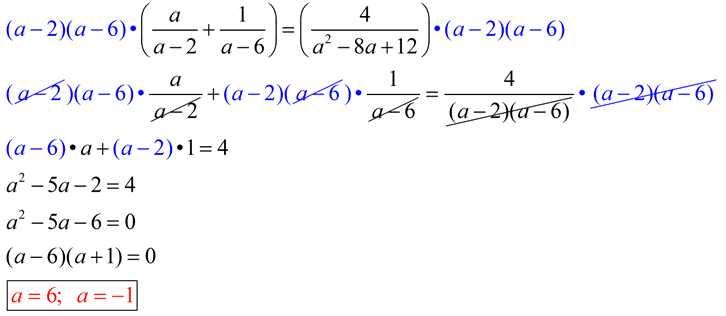## Solving Fractional Equations

Solving fractional equations is very much like addition and subtraction of fractions, but after the first step, you will GET RID of the denominators! “Getting rid” of the denominators is actually changing them all to the value of 1, which of course does not need to be written, leaving us with a denominator-free equation. YEA!!

### To “get rid” of the denominators,

 What to do … Why it works … 1.  start by choosing the common denominator for the equation. Terms in equations are often connected by addition or subtraction.  Dealing with addition or subtraction of fractions requires a common denominator. 2.  multiply EVERY TERM in the equation by the common denominator. In an equation, (unlike an expression), you may multiply “every term” on both sides of the equal sign by the same value and not change the equation.  You maintain a balanced equation. 3.  reduce each term to form a “denominator free” equation. All denominators in the equation can be reduced with our common denominator, thus leaving all equation denominators as a value of one.

Example 1:
1. Determine domain values that will create zero denominators.2. Find the least common denominator.
In this example, the least common denominator is 2x(x + 1) or 2x² + 2x.
3. Multiply both sides of the equation by the least common denominator. Be careful on the right hand side of the equation which contains subtraction. Be sure to distribute across the parentheses (across the subtraction). Reduce, simplify, and solve for x.4. Check to be sure that each answer does not cause a zero denominator problem in the original equation.
From our domain check of the original equation (step 1), we know that the answers of x = -1 and 0 will NOT be solutions. Our solution should be OK.
Show the check:Example 2:
1. Determine domain values that will create zero denominators.2. Find the least common denominator.
In this example, after factoring a² – 8a + 12 into (a – 2)(a – 6), we can see that the least common denominator is a² – 8a + 12 or (a – 2)(a – 6).
3. Multiply both sides of the equation by the least common denominator. Be careful on the left hand side of the equation which contains addition. Be sure to distribute across the parentheses (across the addition). Reduce, simplify, and solve for a.4. Check to be sure that each answer does not cause a zero denominator problem in the original equation.
From our domain check of the original equation (step 1), we know that the answer of a = 6 will NOT be a solution, since it causes a zero denominator problem. The only possible solution is a = -1.
Show the checks: